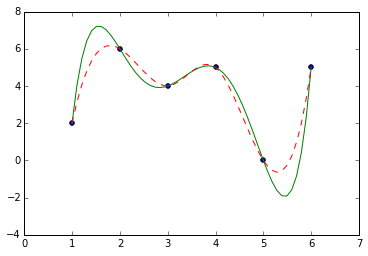# Numerical Linear Algebra 2 and Data Fitting Notes

## Least Squares and QR Factorization

We started with the normal equations $A^t A x = A^b$ and proposed a method via a $QR$-factorization:

$$Rx = Q^t b$$

One thing that a number of people noticed is that they were getting complaints of a singular matrix $R$. The was caused by Scipy using a particular convention for $QR$ factorization.

One way you may implement this is by taking the columns of $A$ and performing something like Gram-Schmidt orthogonalization. The resulting vectors will make up the $Q$ matrix (and have the same shape as $A$). If you're careful and keep track of the coefficients relating the columns of $A$ and $Q$, you can organize them into an invertible upper triangular matrix $R$. I think this is sometimes called the reduced or economic $QR$.

We could stop there (which is what I had in mind for this problem). However, you could insist on augmenting your $Q$ matrix with additional columns that span the entire codomain. In this case, to ensure we still have $A = QR$, we'll also need to augment $R$ with some rows of zeros. This is sometimes called the full $QR$.

Notice the different in the following example:

In :
import numpy as np
from scipy.linalg import qr

A = np.random.rand(3, 2)
Q, R = qr(A, mode='economic')

print 'Economic QR'
print
print Q
print
print R
print

A = np.random.rand(3, 2)
Q, R = qr(A, mode='full')

print 'Full QR'
print
print Q
print
print R
print

Economic QR

[[-0.9958786  -0.03565588]
[-0.08201575 -0.03850109]
[-0.03871997  0.99862221]]

[[-0.76981659 -0.92074848]
[ 0.          0.81343184]]

Full QR

[[-0.69505576  0.53518607  0.48007641]
[-0.71082812 -0.41142492 -0.57048481]
[-0.10780013 -0.73777057  0.66638857]]

[[-1.38339218 -0.81672751]
[ 0.         -0.54773086]
[ 0.          0.        ]]



This wasn't an issue before since on an invertible square matrix, both conventions agree.

## Built-in Polynomial and Spline Interpolation Interfaces

Since it's a lot of random stuff to remember, I wanted to put a reference to the polynomial and spline interpolation interfaces I used for the demonstrations today.

In :
%matplotlib inline
import matplotlib.pyplot as plt
from scipy.interpolate import InterpolatedUnivariateSpline as Spline

x = np.array([1., 2., 3., 4., 5., 6.])
y = np.array([2., 6., 4., 5., 0., 5.])
p = np.poly1d(np.polyfit(x, y, 5)) # <- polyfit gives you the points and poly1d turns it into a convinient object for evaluation.
spl = Spline(x, y)

t = np.linspace(1, 6, 50)

plt.figure()
plt.plot(t, p(t), 'g-')
plt.plot(t, spl(t), 'r--')
plt.scatter(x, y)
plt.show()